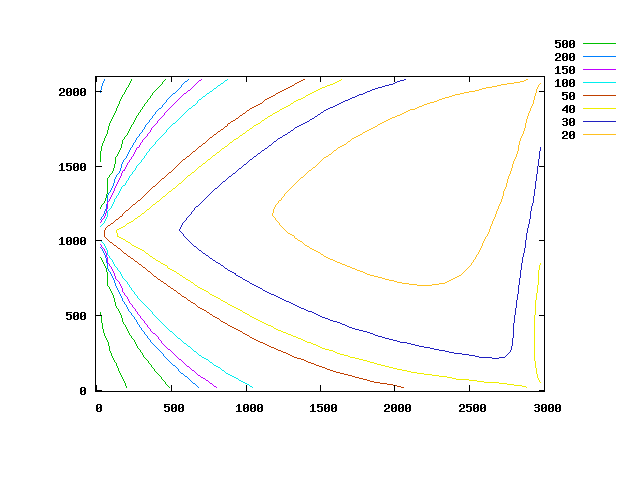# Beacon system using triangulation (from robot)

To find its position, a sensor is attached to a robot which measures angles between beacons attached at playground beacon supports.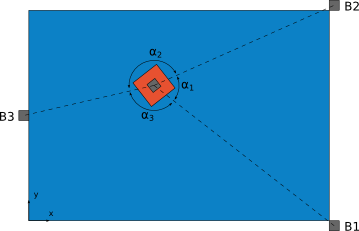Beacon B1 is at (3000, 0), B2 at (3000, 2100), B3 at (0, 1050).

## Precision evaluation

In the following graphs, `angle_b`nm is the angle measured between Bn and Bm. A resolution of one degree has been assumed. The lines represents the set of positions for which the angle is the same.

First, here is the found angles according to robot position: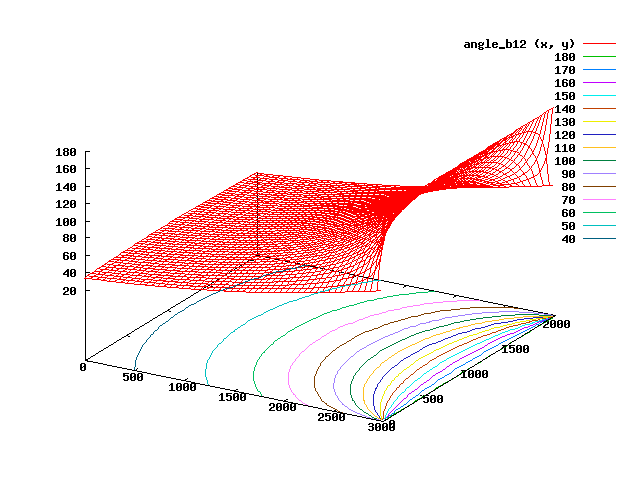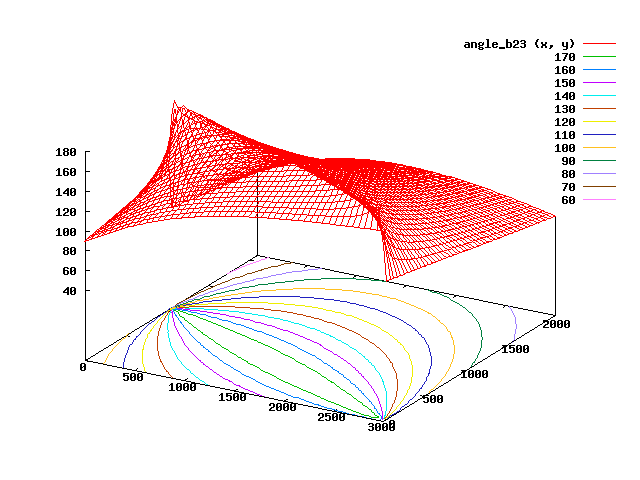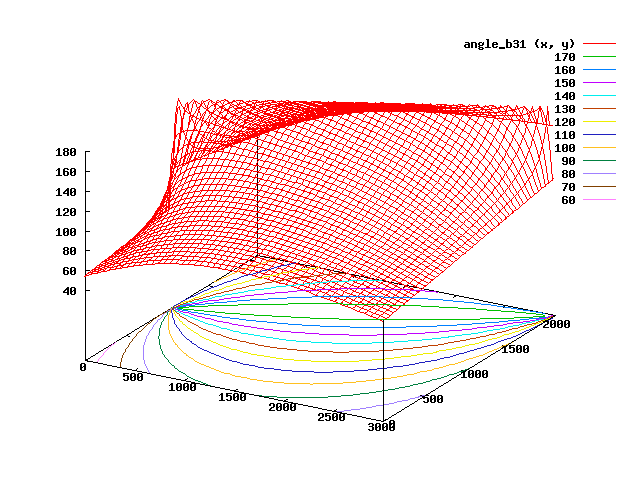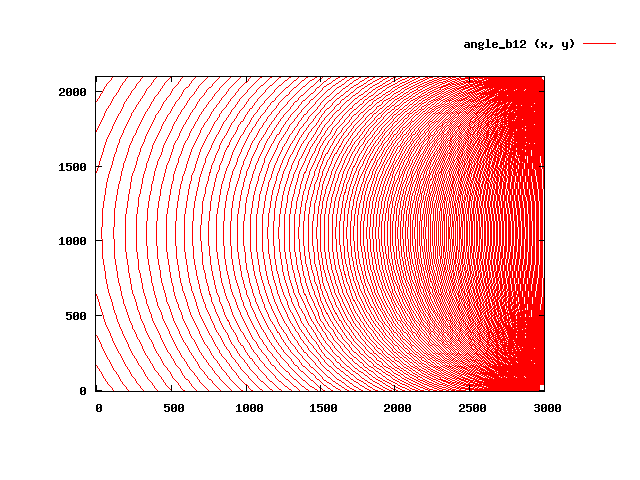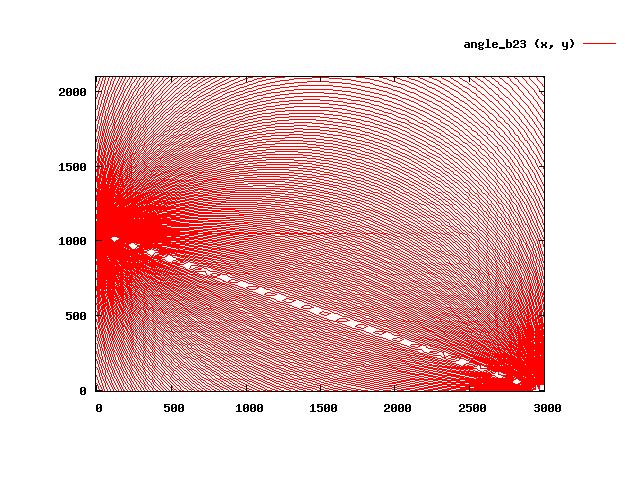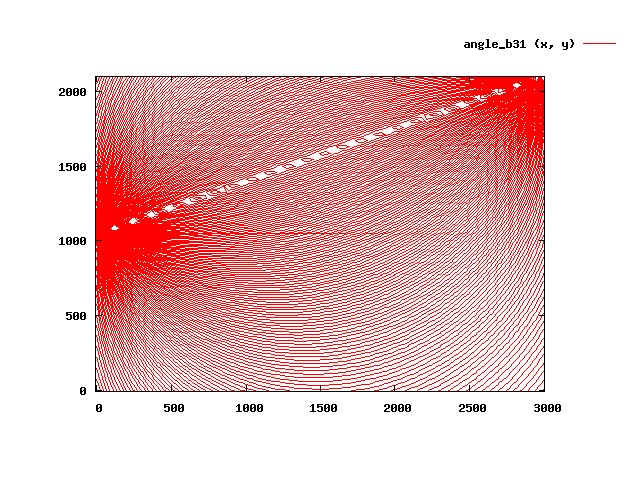With all three beacon, quite unreadable: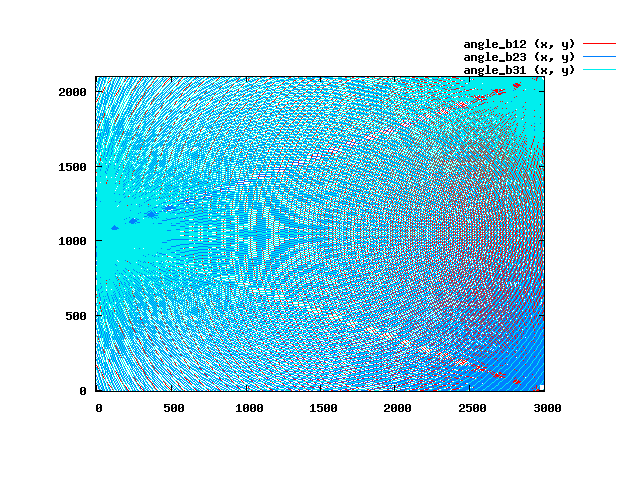Same thing, but with 3 degree of resolution: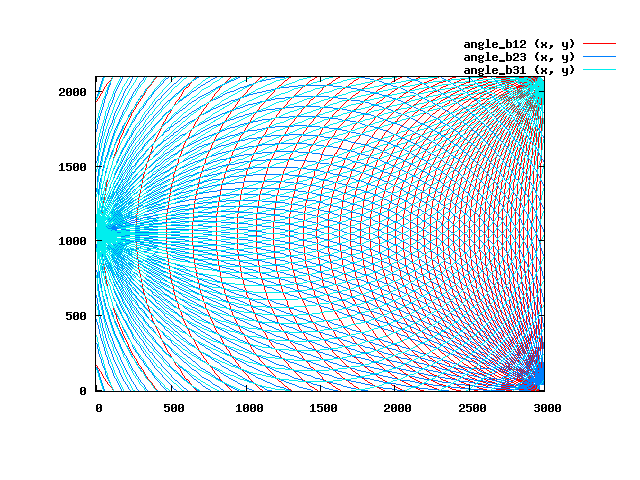Zoom to some interresting regions, each square is 10 cm wide (back to 1 degree of resolution):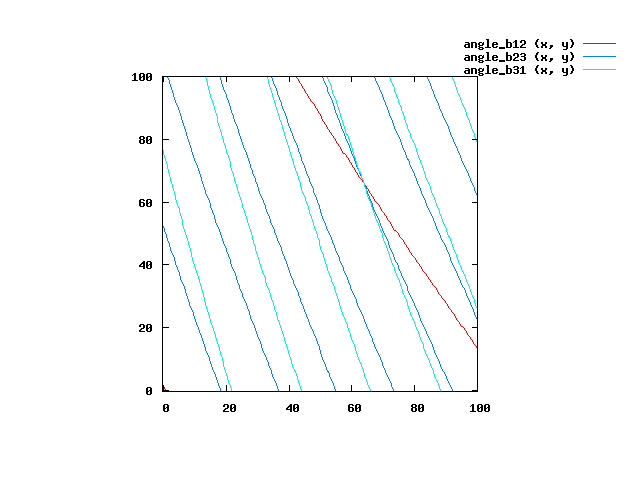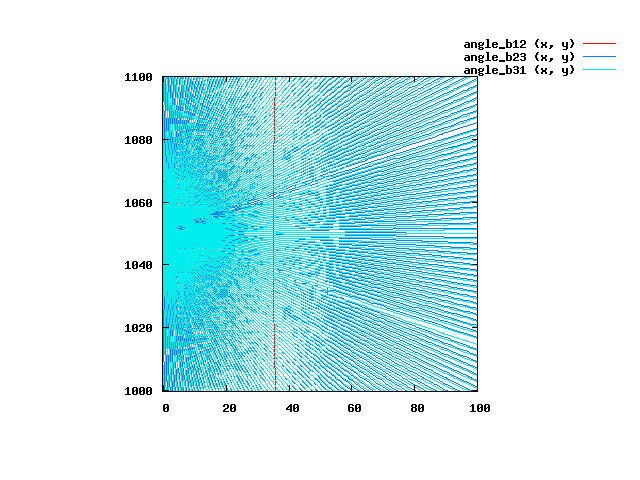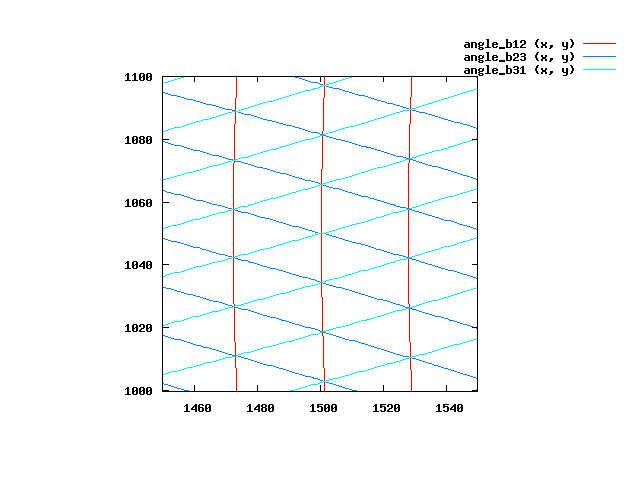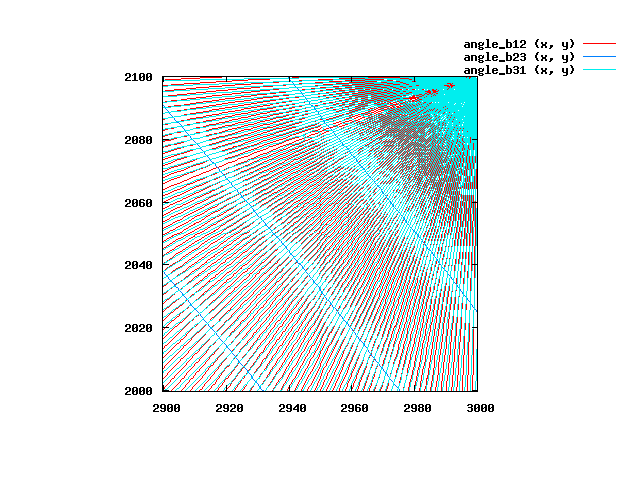Evaluation of position error: